Next: About this document ... Up: lab_template Previous: lab_template

Subsections

# Solids of Revolution

## Introduction

The purpose of this lab is to use Maple to study solids of revolution. Solids of revolution are created by rotating curves in the x-y plane about an axis, generating a three dimensional object.

## Background

So far we have used the integral mainly to to compute areas of plane regions. It turns out that the definite integral can also be used to calculate the volumes of certain types of three-dimensional solids. The class of solids we will consider in this lab are called Solids of Revolution because they can be obtained by revolving a plane region about an axis.

As a simple example, consider the graph of the function
for
, which appears below.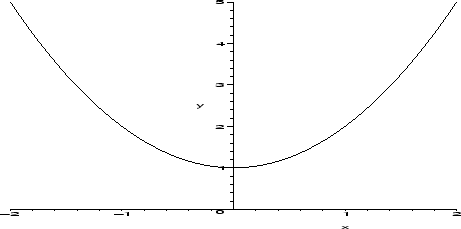If we take the region between the graph and the x-axis and revolve it about the x-axis, we obtain the solid pictured in the next graph.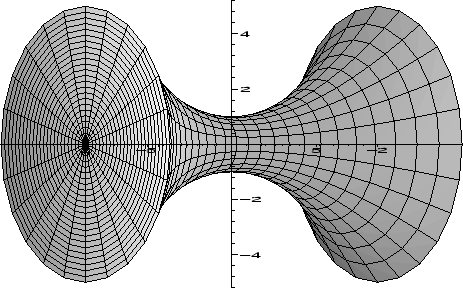To help you in plotting surfaces of revolution, A Maple procedure called revolve has been written. The commands used to produce the graphs are shown below. The revolve procedure, as well as the RevInt, LeftInt, and LeftDisk procedures described below are all part of the CalcP7 package, which must be loaded first. The last line in the example below shows the optional argument for revolving the graph ofabout the line
instead of the default.

> with(CalcP7):
> f := x -> x^2+1;
> plot(f(x),x=-2..2,y=0..5);
> revolve(f(x),x=-2..2);
> revolve(f(x),x=-2..2,y=-2);


The revolve command has other options that you should read about in the help screen. For example, you can speed the command up by only plotting the surface generated by revolving the curve with the nocap argument, and you can also plot a solid of revolution formed by revolving the area between two functions. Try the following examples. (Note: The last example shows how to use revolve with a piecewise defined function using the piecewise command.)

> revolve({f(x),0.5},,x=-2..2,y=-1);
> revolve(cos(x),x=0..4*Pi,y=-2,nocap);
> revolve({5,x^2+1},,x=-2..2);
> g := x-> piecewise(x<0,-x+1/2,x^2-x+1/2);
> revolve(g(x),x=-1..2);


It turns out that the volume of the solid obtained by revolving the region between the graph and the
-axis about the-axis can be determined from the integral
to have the value. More generally, if you revolve the area under the graph offor
about the-axis, the volume is given by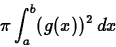Where does this formula come from? To help you understand it, two more Maple procedures, RevInt and LeftDisk, have been written. The procedure RevInt sets up the integral for the volume of a solid of revolution as shown below. The Maple commands evalf and value can be used to obtain a numerical or analytical value.

The integral formula given above for the volume of a solid of revolution comes, as usual, from a limit process. Recall the rectangular approximations we used for plane regions. If you think of taking one of the rectangles and revolving it about the x-axis, you get a disk whose radius is the heightof the rectangle and thickness is
, the width of the rectangle. The volume of this disk is. If you revolve all of the rectangles in the rectangular approximation about the x-axis, you get a solid made up of disks that approximates the volume of the solid of revolution obtained by revolving the plane region about the x-axis.

To help you visualize this approximation of the volume by disks, the LeftDisk procedure has been written. The syntax for this command is similar to that for revolve, except that the number of subintervals must be specified. The examples below produce approximations with five and ten disks. The latter approximation is shown in the graph below.

> LeftDisk(f(x),x=-2..2,5);
> LeftDisk(f(x),x=-2..2,10);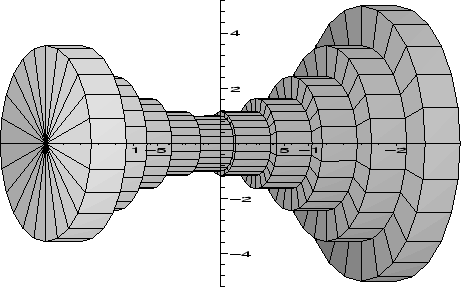## Finding Volumes of Revolution

In order to calculate the volume of a solid of revolution, you can either use the int command implementing the formula above or use the Maple procedure RevInt which sets up the integral for you. Try the examples below to see the different types of output.

> Pi*int(f(x)^2,x=-2..2);
> evalf(Pi*int(f(x)^2,x=-2..2));
> RevInt(f(x),x=-2..2);
> value(RevInt(f(x),x=-2..2));
> evalf(RevInt(f(x),x=-2..2));


## Exercises

1. For the function
over the interval
,
A
Plotover the given interval.
B
Plot the approximation of the solid of revolution using LeftDisk with 12 disks.
C
Plot the solid formed by revolvingabout the-axis.
D
Plot the solid formed by revolvingabout the line
.
E
Find the exact volume of the solid of revolution using the RevInt command and label your output exact.
F
Find the number of subintervals needed to approximate the volume of the solid of revolution about the-axis using LeftInt with error no greater than 0.1.

2. A brass finial is to be made in the shape of the solid obtained by revolving the functionabout theaxis over the interval. (A finial is a decorative cap or projection often seen on top of fence posts or staircase posts.) If the dimensions of the solid are all in inches, determine how many cubic inches of brass will be needed to make
of these finials.Next: About this document ... Up: lab_template Previous: lab_template
Jane E Bouchard
2005-03-03